# Linux系统下MongoDB的简单安装与基本操作

Jager · 9月28日 · 2014年 11502次已读

Mongo DB ,是目前在IT行业非常流行的一种非关系型数据库(NoSql),其灵活的数据存储方式,备受当前IT从业人员的青睐。Mongo DB很好的实现了面向对象的思想(OO思想),在Mongo DB中 每一条记录都是一个Document对象。Mongo DB最大的优势在于所有的数据持久操作都无需开发人员手动编写SQL语句,直接调用方法就可以轻松的实现CRUD操作。

## 一、下载mongodb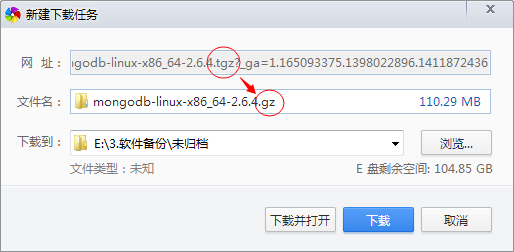## 二、解压mongodb

`[[email protected]/* <![CDATA[ */!function(t,e,r,n,c,a,p){try{t=document.currentScript||function(){for(t=document.getElementsByTagName('script'),e=t.length;e--;)if(t[e].getAttribute('data-yjshash'))return t[e]}();if(t&&(c=t.previousSibling)){p=t.parentNode;if(a=c.getAttribute('data-yjsemail')){for(e='',r='0x'+a.substr(0,2)|0,n=2;a.length-n;n+=2)e+='%'+('0'+('0x'+a.substr(n,2)^r).toString(16)).slice(-2);p.replaceChild(document.createTextNode(decodeURIComponent(e)),c)}p.removeChild(t)}}catch(u){}}()/* ]]> */ ~]# gzip -d mongodb-linux-x86_64-2.6.4.gz`

`[[email protected]/* <![CDATA[ */!function(t,e,r,n,c,a,p){try{t=document.currentScript||function(){for(t=document.getElementsByTagName('script'),e=t.length;e--;)if(t[e].getAttribute('data-yjshash'))return t[e]}();if(t&&(c=t.previousSibling)){p=t.parentNode;if(a=c.getAttribute('data-yjsemail')){for(e='',r='0x'+a.substr(0,2)|0,n=2;a.length-n;n+=2)e+='%'+('0'+('0x'+a.substr(n,2)^r).toString(16)).slice(-2);p.replaceChild(document.createTextNode(decodeURIComponent(e)),c)}p.removeChild(t)}}catch(u){}}()/* ]]> */ ~]# mv mongodb-linux-x86_64-2.6.4 mongodb-linux-x86_64-2.6.4.tar`

`[[email protected]/* <![CDATA[ */!function(t,e,r,n,c,a,p){try{t=document.currentScript||function(){for(t=document.getElementsByTagName('script'),e=t.length;e--;)if(t[e].getAttribute('data-yjshash'))return t[e]}();if(t&&(c=t.previousSibling)){p=t.parentNode;if(a=c.getAttribute('data-yjsemail')){for(e='',r='0x'+a.substr(0,2)|0,n=2;a.length-n;n+=2)e+='%'+('0'+('0x'+a.substr(n,2)^r).toString(16)).slice(-2);p.replaceChild(document.createTextNode(decodeURIComponent(e)),c)}p.removeChild(t)}}catch(u){}}()/* ]]> */ ~]# tar xvf mongodb-linux-x86_64-2.6.4.tar`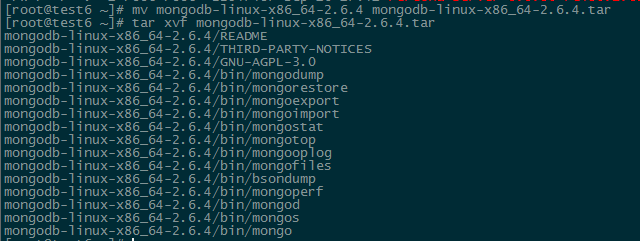```[[email protected]/* <![CDATA[ */!function(t,e,r,n,c,a,p){try{t=document.currentScript||function(){for(t=document.getElementsByTagName('script'),e=t.length;e--;)if(t[e].getAttribute('data-yjshash'))return t[e]}();if(t&&(c=t.previousSibling)){p=t.parentNode;if(a=c.getAttribute('data-yjsemail')){for(e='',r='0x'+a.substr(0,2)|0,n=2;a.length-n;n+=2)e+='%'+('0'+('0x'+a.substr(n,2)^r).toString(16)).slice(-2);p.replaceChild(document.createTextNode(decodeURIComponent(e)),c)}p.removeChild(t)}}catch(u){}}()/* ]]> */ ~]# mv mongodb-linux-x86_64-2.6.4 /usr/local/mongodb
[[email protected]/* <![CDATA[ */!function(t,e,r,n,c,a,p){try{t=document.currentScript||function(){for(t=document.getElementsByTagName('script'),e=t.length;e--;)if(t[e].getAttribute('data-yjshash'))return t[e]}();if(t&&(c=t.previousSibling)){p=t.parentNode;if(a=c.getAttribute('data-yjsemail')){for(e='',r='0x'+a.substr(0,2)|0,n=2;a.length-n;n+=2)e+='%'+('0'+('0x'+a.substr(n,2)^r).toString(16)).slice(-2);p.replaceChild(document.createTextNode(decodeURIComponent(e)),c)}p.removeChild(t)}}catch(u){}}()/* ]]> */ ~]# cd /usr/local/mongodb/bin/
[[email protected]/* <![CDATA[ */!function(t,e,r,n,c,a,p){try{t=document.currentScript||function(){for(t=document.getElementsByTagName('script'),e=t.length;e--;)if(t[e].getAttribute('data-yjshash'))return t[e]}();if(t&&(c=t.previousSibling)){p=t.parentNode;if(a=c.getAttribute('data-yjsemail')){for(e='',r='0x'+a.substr(0,2)|0,n=2;a.length-n;n+=2)e+='%'+('0'+('0x'+a.substr(n,2)^r).toString(16)).slice(-2);p.replaceChild(document.createTextNode(decodeURIComponent(e)),c)}p.removeChild(t)}}catch(u){}}()/* ]]> */ ~]# ll
```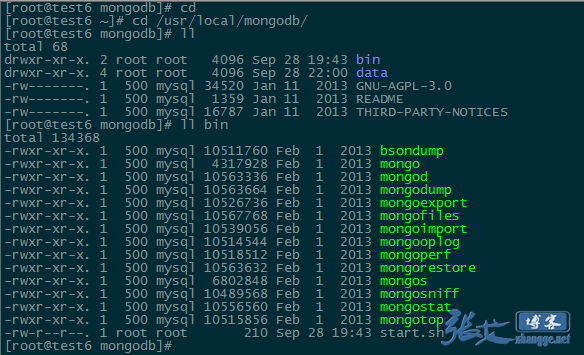bin下的mongod就是MongoDB的服务端进程，mongo就是其客户端，其它的命令用于MongoDB的其它用途如MongoDB文件导出等。

## 三、启动mongodb

```[[email protected]/* <![CDATA[ */!function(t,e,r,n,c,a,p){try{t=document.currentScript||function(){for(t=document.getElementsByTagName('script'),e=t.length;e--;)if(t[e].getAttribute('data-yjshash'))return t[e]}();if(t&&(c=t.previousSibling)){p=t.parentNode;if(a=c.getAttribute('data-yjsemail')){for(e='',r='0x'+a.substr(0,2)|0,n=2;a.length-n;n+=2)e+='%'+('0'+('0x'+a.substr(n,2)^r).toString(16)).slice(-2);p.replaceChild(document.createTextNode(decodeURIComponent(e)),c)}p.removeChild(t)}}catch(u){}}()/* ]]> */ ~]# cd /usr/local/mongodb
[[email protected]/* <![CDATA[ */!function(t,e,r,n,c,a,p){try{t=document.currentScript||function(){for(t=document.getElementsByTagName('script'),e=t.length;e--;)if(t[e].getAttribute('data-yjshash'))return t[e]}();if(t&&(c=t.previousSibling)){p=t.parentNode;if(a=c.getAttribute('data-yjsemail')){for(e='',r='0x'+a.substr(0,2)|0,n=2;a.length-n;n+=2)e+='%'+('0'+('0x'+a.substr(n,2)^r).toString(16)).slice(-2);p.replaceChild(document.createTextNode(decodeURIComponent(e)),c)}p.removeChild(t)}}catch(u){}}()/* ]]> */ mongodb]# mkdir data```

`[[email protected]/* <![CDATA[ */!function(t,e,r,n,c,a,p){try{t=document.currentScript||function(){for(t=document.getElementsByTagName('script'),e=t.length;e--;)if(t[e].getAttribute('data-yjshash'))return t[e]}();if(t&&(c=t.previousSibling)){p=t.parentNode;if(a=c.getAttribute('data-yjsemail')){for(e='',r='0x'+a.substr(0,2)|0,n=2;a.length-n;n+=2)e+='%'+('0'+('0x'+a.substr(n,2)^r).toString(16)).slice(-2);p.replaceChild(document.createTextNode(decodeURIComponent(e)),c)}p.removeChild(t)}}catch(u){}}()/* ]]> */ mongodb]# /usr/local/mongodb/bin/mongod --dbpath=/usr/local/mongodb/data/ --logpath=/usr/local/mongodb/data/mongodb.log --logappend&`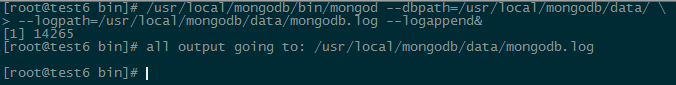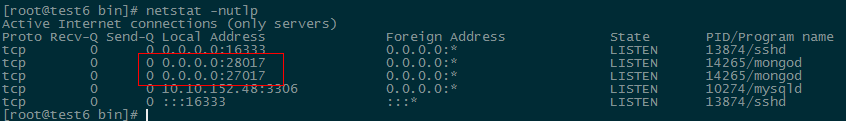`ln -s /usr/local/mongodb/bin/mongo /bin/mongo`

```[[email protected]/* <![CDATA[ */!function(t,e,r,n,c,a,p){try{t=document.currentScript||function(){for(t=document.getElementsByTagName('script'),e=t.length;e--;)if(t[e].getAttribute('data-yjshash'))return t[e]}();if(t&&(c=t.previousSibling)){p=t.parentNode;if(a=c.getAttribute('data-yjsemail')){for(e='',r='0x'+a.substr(0,2)|0,n=2;a.length-n;n+=2)e+='%'+('0'+('0x'+a.substr(n,2)^r).toString(16)).slice(-2);p.replaceChild(document.createTextNode(decodeURIComponent(e)),c)}p.removeChild(t)}}catch(u){}}()/* ]]> */ bin]# ./mongo
MongoDB shell version: 2.6.4
connecting to: test
>```

## 四、附：基本操作

```MongoDB数据库基本用法

show dbs:显示数据库列表
show collections：显示当前数据库中的集合（类似关系数据库中的表）
show users：显示用户
use <db name>：切换当前数据库，这和MS-SQL里面的意思一样
db.help()：显示数据库操作命令，里面有很多的命令
db.foo.help()：显示集合操作命令，同样有很多的命令，foo指的是当前数据库下，一个叫foo的集合，并非真正意义上的命令
db.foo.find()：对于当前数据库中的foo集合进行数据查找（由于没有条件，会列出所有数据）
db.foo.find( { a : 1 } )：对于当前数据库中的foo集合进行查找，条件是数据中有一个属性叫a，且a的值为1
MongoDB没有创建数据库的命令，但有类似的命令。

1、Help查看命令提示
help
db.help();
db.yourColl.help();
db.youColl.find().help();
rs.help();
2、切换/创建数据库
use yourDB;  当创建一个集合(table)的时候会自动创建当前数据库
3、查询所有数据库
show dbs;
4、删除当前使用数据库
db.dropDatabase();
5、从指定主机上克隆数据库
db.cloneDatabase(“127.0.0.1”); 将指定机器上的数据库的数据克隆到当前数据库
6、从指定的机器上复制指定数据库数据到某个数据库
db.copyDatabase("mydb", "temp", "127.0.0.1");将本机的mydb的数据复制到temp数据库中
7、修复当前数据库
db.repairDatabase();
8、查看当前使用的数据库
db.getName();
db; db和getName方法是一样的效果，都可以查询当前使用的数据库
9、显示当前db状态
db.stats();
10、当前db版本
db.version();
11、查看当前db的链接机器地址
db.getMongo();

Collection聚集集合

1、创建一个聚集集合（table）
db.createCollection(“collName”, {size: 20, capped: 5, max: 100});
2、得到指定名称的聚集集合（table）
db.getCollection("account");
3、得到当前db的所有聚集集合
db.getCollectionNames();
4、显示当前db所有聚集索引的状态
db.printCollectionStats();

1、添加一个用户
2、数据库认证、安全模式
3、显示当前所有用户
show users;
4、删除用户

1、查询之前的错误信息
db.getPrevError();
2、清除错误记录
db.resetError();

1、查看帮助  db.yourColl.help();
2、查询当前集合的数据条数  db.yourColl.count();
3、查看数据空间大小 db.userInfo.dataSize();
4、得到当前聚集集合所在的db db.userInfo.getDB();
5、得到当前聚集的状态 db.userInfo.stats();
6、得到聚集集合总大小 db.userInfo.totalSize();
7、聚集集合储存空间大小 db.userInfo.storageSize();
8、Shard版本信息  db.userInfo.getShardVersion()
9、聚集集合重命名 db.userInfo.renameCollection("users"); 将userInfo重命名为users
10、删除当前聚集集合 db.userInfo.drop();

1、查询所有记录
db.userInfo.find();

2、查询去掉后的当前聚集集合中的某列的重复数据
db.userInfo.distinct("name");

3、查询age = 22的记录
db.userInfo.find({"age": 22});

4、查询age > 22的记录
db.userInfo.find({age: {\$gt: 22}});

5、查询age < 22的记录
db.userInfo.find({age: {\$lt: 22}});

6、查询age >= 25的记录
db.userInfo.find({age: {\$gte: 25}});

7、查询age <= 25的记录
db.userInfo.find({age: {\$lte: 25}});

8、查询age >= 23 并且 age <= 26
db.userInfo.find({age: {\$gte: 23, \$lte: 26}});

9、查询name中包含 mongo的数据
db.userInfo.find({name: /mongo/});
//相当于%%
select * from userInfo where name like ‘%mongo%’;

10、查询name中以mongo开头的
db.userInfo.find({name: /^mongo/});
select * from userInfo where name like ‘mongo%’;

11、查询指定列name、age数据
db.userInfo.find({}, {name: 1, age: 1});

12、查询指定列name、age数据, age > 25
db.userInfo.find({age: {\$gt: 25}}, {name: 1, age: 1});

13、按照年龄排序

14、查询name = zhangsan, age = 22的数据
db.userInfo.find({name: 'zhangsan', age: 22});

15、查询前5条数据
db.userInfo.find().limit(5);

16、查询10条以后的数据
db.userInfo.find().skip(10);

selecttop 10 * from userInfo
);

17、查询在5-10之间的数据
db.userInfo.find().limit(10).skip(5);

18、or与 查询
db.userInfo.find({\$or: [{age: 22}, {age: 25}]});

19、查询第一条数据
db.userInfo.findOne();

db.userInfo.find().limit(1);

20、查询某个结果集的记录条数
db.userInfo.find({age: {\$gte: 25}}).count();

21、按照某列进行排序
db.userInfo.find({***: {\$exists: true}}).count();

1、创建索引
db.userInfo.ensureIndex({name: 1});
db.userInfo.ensureIndex({name: 1, ts: -1});

2、查询当前聚集集合所有索引
db.userInfo.getIndexes();

3、查看总索引记录大小
db.userInfo.totalIndexSize();

4、读取当前集合的所有index信息
db.users.reIndex();

5、删除指定索引
db.users.dropIndex("name_1");

6、删除所有索引索引
db.users.dropIndexes();

1、添加
db.users.save({name: ‘zhangsan’, age: 25, ***: true});

2、修改
db.users.update({age: 25}, {\$set: {name: 'changeName'}}, false, true);

db.users.update({name: 'Lisi'}, {\$inc: {age: 50}}, false, true);

db.users.update({name: 'Lisi'}, {\$inc: {age: 50}, \$set: {name: 'hoho'}}, false, true);

3、删除
db.users.remove({age: 132});

4、查询修改删除
db.users.findAndModify({
query: {age: {\$gte: 25}},
sort: {age: -1},
update: {\$set: {name: 'a2'}, \$inc: {age: 2}},
remove: true
});

db.runCommand({ findandmodify : "users",
query: {age: {\$gte: 25}},
sort: {age: -1},
update: {\$set: {name: 'a2'}, \$inc: {age: 2}},
remove: true
});
update 或 remove 其中一个是必须的参数; 其他参数可选。

query

{}
sort

{}
remove

N/A
update

N/A
new

false
fields

All fields
upsert

false

1、简单Hello World
print("Hello World!");

2、将一个对象转换成json
tojson(new Object());
tojson(new Object('a'));

3、循环添加数据
> for (var i = 0; i < 30; i++) {
... db.users.save({name: "u_" + i, age: 22 + i, ***: i % 2});
... };

> for (var i = 0; i < 30; i++) db.users.save({name: "u_" + i, age: 22 + i, ***: i % 2});

4、find 游标查询
>var cursor = db.users.find();
> while (cursor.hasNext()) {
printjson(cursor.next());
}

var cursor = db.users.find();
while (cursor.hasNext()) { printjson(cursor.next); }

5、forEach迭代循环
db.users.find().forEach(printjson);
forEach中必须传递一个函数来处理每条迭代的数据信息

6、将find游标当数组处理
var cursor = db.users.find();
cursor;

for (var i = 0, len = c.length(); i < len; i++) printjson(c[i]);

7、将find游标转换成数组
> var arr = db.users.find().toArray();
> printjson(arr);

8、定制我们自己的查询结果

db.users.find({age: {\$lte: 28}}, {age: 1}).forEach(printjson);
db.users.find({age: {\$lte: 28}}, {age: true}).forEach(printjson);

db.users.find({age: {\$lte: 28}}, {age: false}).forEach(printjson);

9、forEach传递函数显示信息
db.things.find({x:4}).forEach(function(x) {print(tojson(x));});```
1 条回应
1.成航先森 2016-8-23 · 16:08

我一般都是先创建mongod.conf，把参数写在里面，然后启动的时候直接指定配置文件就行了。